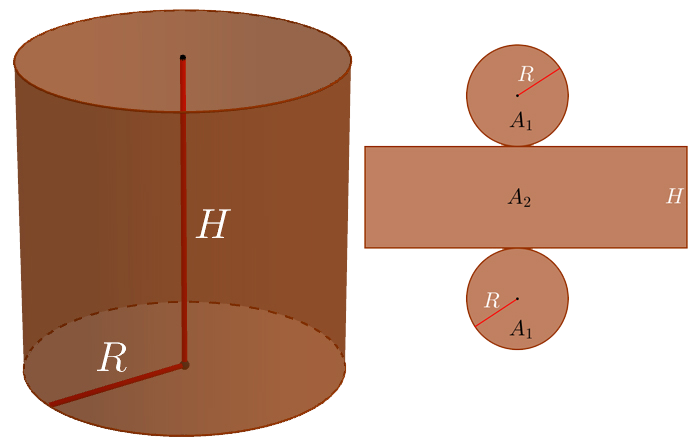# CylinderArea

$A=2{A}_{1}+{A}_{2}=2{R}^{2}\pi +2R\pi H$

Base Area

${A}_{1}={R}^{2}\pi$

Side Area

${A}_{2}=2R\pi H$

Volume

$V={A}_{1}·H={R}^{2}\pi H$
Keywords: cylinder, area, volume